# Redshift

The redshift of a given object is a measure of the amount that the wavelength of its emitted or reflected electromagnetic radiation has increased. This increase in wavelength is prevailingly interpreted as due to the relative motion of a source with respect to an observer; a light emitting source moving away from an observer will have its spectrum shifted toward longer (in optical light, redder) wavelengths. In practice this is analogous to the Doppler shifting of sound waves emitted from a moving source. Light emitted from a source moving towards an observer will experience a blueshift. In the case of redshift, however, most of the redshift is believed to be due not to the object moving through space, but to the expansion of space itself.

## Mathematics

The redshift, Z, of an object as measured by an observer is defined as,where theis the wavelength of light emitted by the object and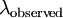is the wavelength measured by the observed. The recessional velocity, v, of an object can be determined from its redshift to be,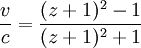where c is the speed of light. At small recessional velocities/redshifts, the formula can be simplified to,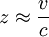## Support for the Big Bang theory

The Hubble redshift in absorption and emission lines in the spectra of distant cosmic objects, discovered by Edwin Hubble in 1929, is claimed as a source of evidence for the Big Bang theory. The redshift, the expanded wavelength of light, suggests that these objects are moving away from Earth due to the expansion of the universe. It also suggests that the universe is undergoing accelerating expansion for an unknown reason.

Actually the reason is known and confirmed by Supernova Cosmology Project team in 1998 with accuracy to one standard deviation (the second term of Taylor series of Hubble "constant" as function of time is causing the illusion of accelerating expansion of space with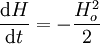,

where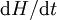is apparent acceleration of expansion of space,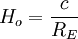,

is Hubble constant, c is speed of light in vacuum, and RE is "Einstein radius", or radius of curvature of space, about 13 billion light years).

## Alternative Interpretations of the Redshift

The redshift phenomenon pertains to observation of spectra of light emitted from luminous bodies such as nebulae (stellar systems). The true nature of light is however still uncertain. With current body of knowledge, the light can be portrayed as out-spreading electromagnetic wave or as travelling in bullet-like parcels of energy called quanta. Waves have various wavelengths; quanta carry different amounts of energy. Since the same phenomenon can be described by both concepts, it is regarded as evidence that there is some underlying connection between them. This connection is expressed in the very fundamental relation between the energy-content of a quantum and the equivalent wavelength:

energy x wavelength = constantBecause of this relation, two possible effects can be identified:

• a.) Increase of wavelengthimplies a decrease in Energy E; If the primary change is in wavelength, then the red-shifts are probably velocity-shifts, what is nowadays prevailing interpretation.
• b.) Reduction of Energy E implies an increase of wavelength; The primary change might be also a loss of energy, which would be observed as redshift.

As in either case it is only increased wavelength what is observed, there is no direct way of determining which of the two above effects is fundamental. In order to be able to distinguish between two alternatives, a critical empirical test is needed that would establish whether or not a luminous body is receding.